# 情人节来了，教你个用Python表白的技巧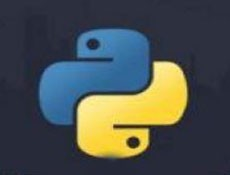• silencement   2020-02-14 13:09:041544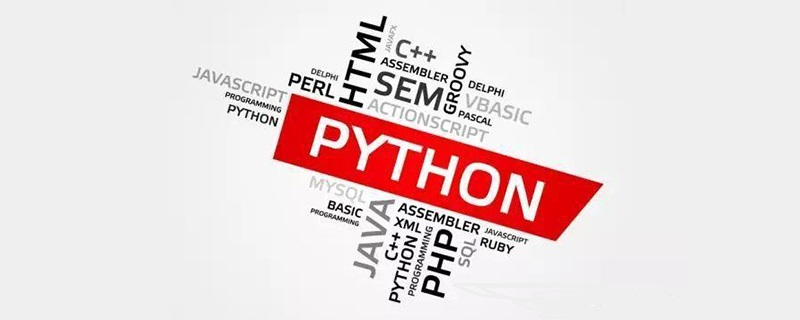2020年，这个看起来如此浪漫的年份，你还是一个人吗？

`难不成我还能是一条狗？`

18年的时候，写过一篇介绍如何使用 Python 来表白的文章。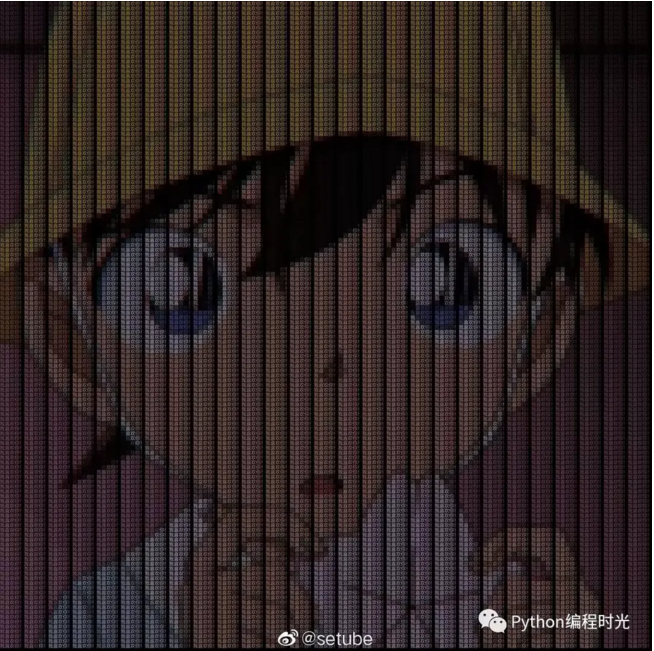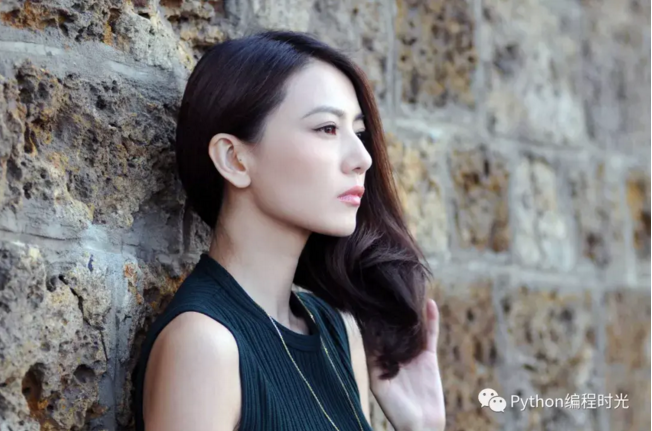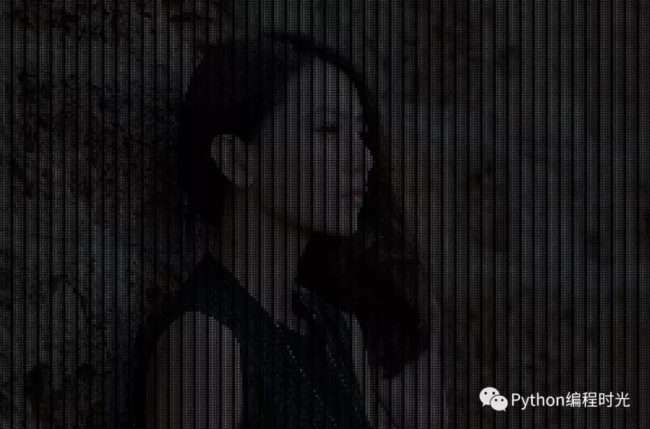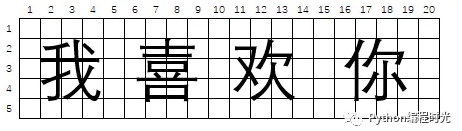```img_raw = Image.open(img_path)

```img_new = Image.new("RGB", img_raw.size, (0, 0, 0))
draw = ImageDraw.Draw(img_new)
font = ImageFont.truetype('C:/Windows/fonts/Dengl.ttf', font_size)```

```def character_generator(text):
while True:
for i in range(len(text)):
yield text[i]```

```for y in range(0, img_raw.size, font_size):
for x in range(0, img_raw.size, font_size):
draw.text((x, y), next(ch_gen), font=font, fill=img_array[x, y], direction=None)```

`img_new.convert('RGB').save("F://gyy_save.jpeg")`

```from PIL import Image, ImageDraw, ImageFont

font_size = 7
text = "我喜欢你！"
img_path = "F://gyy.jpeg"

img_raw = Image.open(img_path)

img_new = Image.new("RGB", img_raw.size, (0, 0, 0))
draw = ImageDraw.Draw(img_new)
font = ImageFont.truetype('C:/Windows/fonts/Dengl.ttf', font_size)

def character_generator(text):
while True:
for i in range(len(text)):
yield text[i]

ch_gen = character_generator(text)

for y in range(0, img_raw.size, font_size):
for x in range(0, img_raw.size, font_size):
draw.text((x, y), next(ch_gen), font=font, fill=img_array[x, y], direction=None)

img_new.convert('RGB').save("F://save.jpeg")```